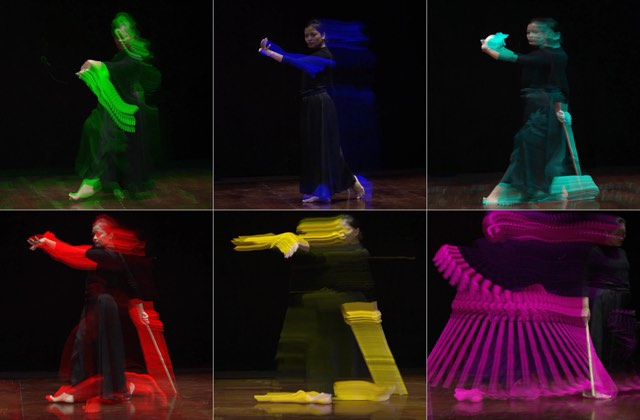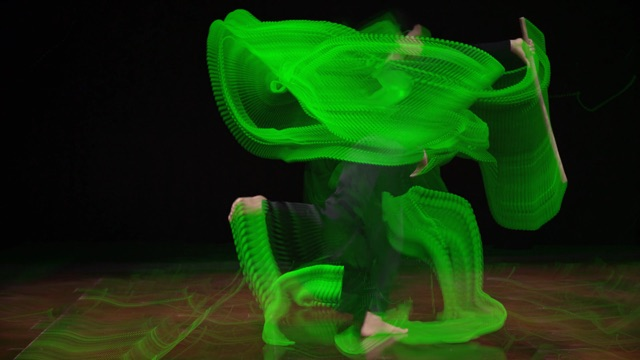"lagfun", what does 'decay', 'planes' do?
+ a little "tmix"

Using a 10 second clip of a dancer, with slow movement on a dark backgronund. This is after testing many alternatives:

planes=7 decay=0.97

ffmpeg lagfun filter, not much information.

From FFmpeg_Book.pdf, 2.52 Lagfun filter, page 76 (version April 4, 2021)
Using a command like this (on my Mac)
`\$ ffmpeg -i in/20210313_01_p_10.mp4 -vf "format=gbrp,lagfun=decay=0.97:planes=1" out/planes=1_97.mp4`planes=1, 2, 3, 4, 5, 6 decay=0.97 (at end of video).planes=1 decay=1.00 at 10 s. This can work with a linear movement across the screen, with a non-moving camera.

"format=gbrp" is not web friendly so to show it on this page I do:
`\$ ffmpeg -i in/planes=7_97.mp4 -vf "format=yuv420p,scale='nhd'" out/s_planes=7_97.mp4`
To see pixel formats in ffmpeg open "ffmpeg -pix_fmts" in Terminal.
ffmpeg Video size.
scale='nhd' 2.4 Video size, 'nhd' 640x360 px

Allowed range for planes is from 0 to 15
planes=0 none effect
planes=1 green
planes=2 blue
planes=3 cyan
planes=4 red
planes=5 yellow
planes=6 magenta
planes=7 white (here more like skin colour)
planes=8=0 none effect
planes=9=1 green
planes=10=2 blue
planes=11=3 cyan
planes=12=4 red
planes=13=5 yellow
planes=14=6 magenta
planes=15=7 white

ffmpeg tmix
If the "trails" of the frames are too distinct the "tmix" filter can mix frames before the "lagfun" filter.
`\$ ffmpeg -i in.mp4 -vf tmix=frames=8:weights="1 1 1 1 1 1 1 1" out_tmix.mp4`

Alexander Refsum Jensenius has worked more on this

tmix + lagfun planes=7 decay=0.97